Function Repository Resource:

# Triangle3DTo2D

Given a 3D triangle, return an equivalent 2D triangle along with a transformation function

Contributed by: Ed Pegg Jr
 Triangle3Dto2D[triangle] computes a two-dimensional triangle along with a rotation matrix and offset vector so that one can recover triangle given in three-dimensional space. Triangle3Dto2D[triangle,"TransformationFunction"] computes a two-dimensional triangle along with a TransformationFunction so that one can recover triangle given in three-dimensional space.

## Examples

### Basic Examples (2)

Given a 3D triangle, generate a 2D triangle, a matrix and an offset:

 In:=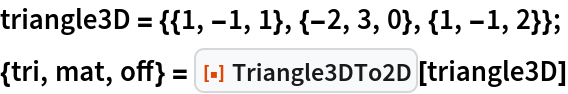Out=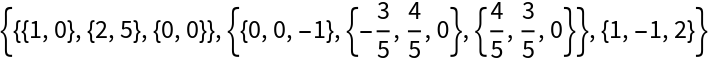In:=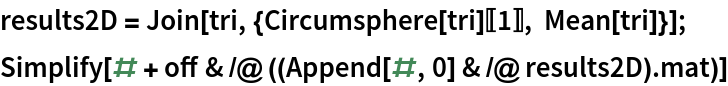Out=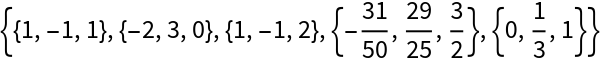### Scope (2)

Generate a 2D triangle and a transformation function from a 3D triangle:

 In:=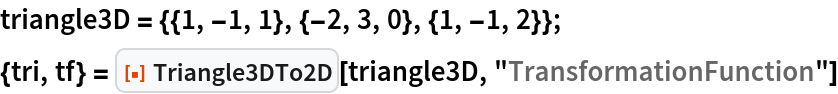Out=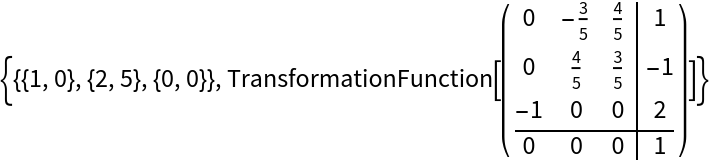In:=Out=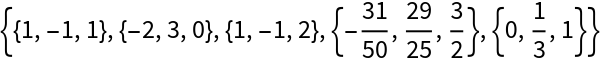The matrix and offset can be discarded:

 In:=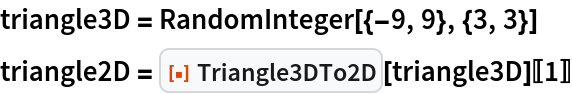Out=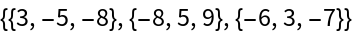Out=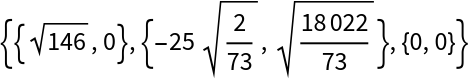Show both triangles in a 3D graphic:

 In:=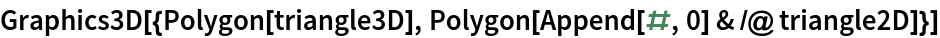Out=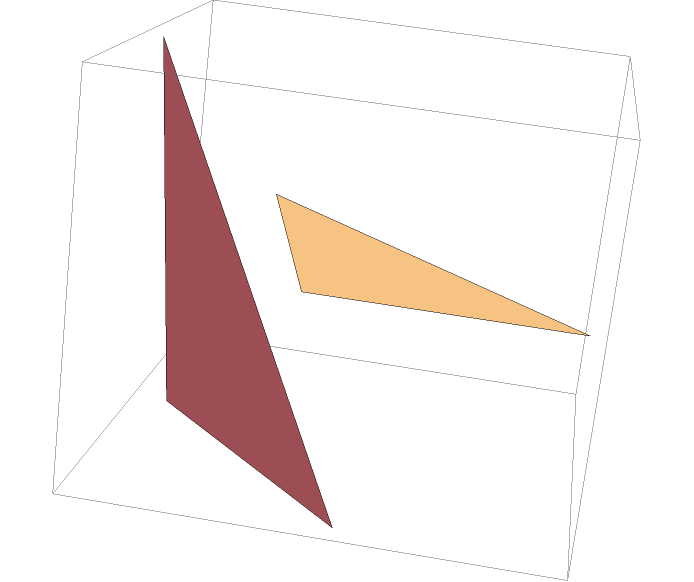### Neat Examples (3)

Find triangles of a polyhedron:

 In:=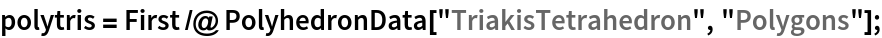Look at the 2D triangle for one of them:

 In:=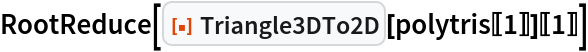Out=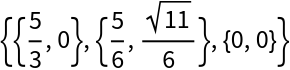Draw tangent spheres on each triangle:

 In:=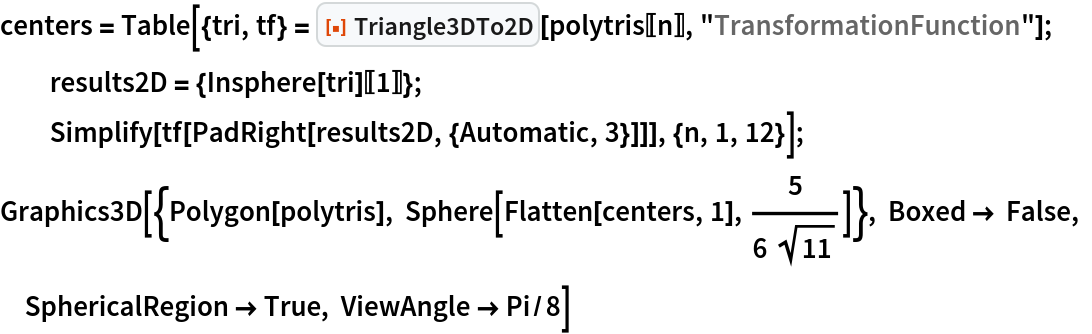Out=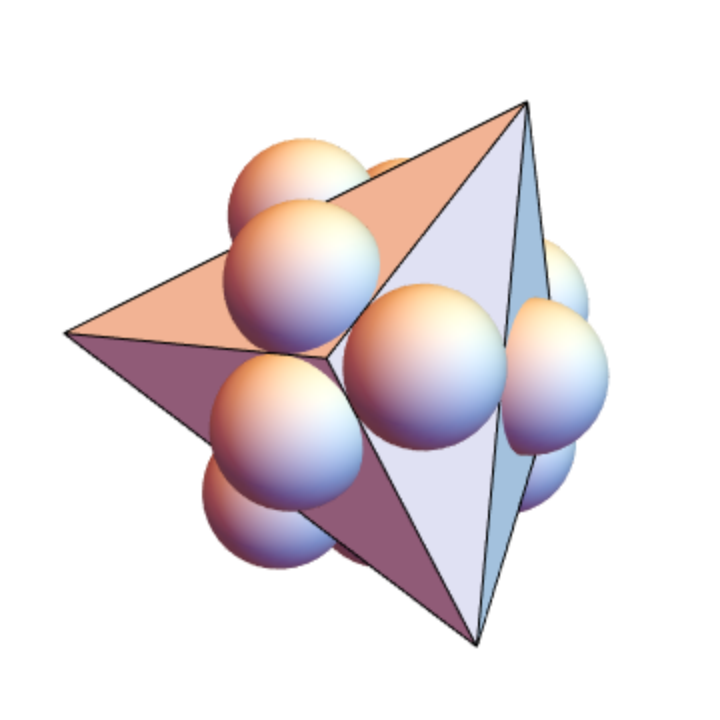## Version History

• 1.1.0 – 14 June 2021
• 1.0.0 – 21 October 2019